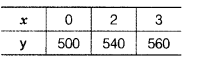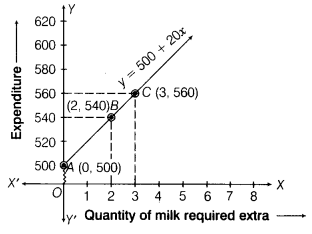# A part of monthly expenses of a family on milk is fixed which is ₹ 500

A part of monthly expenses of a family on milk is fixed which is ₹ 500 and the remaining varies with the quantity of milk taken extra at the rate of ₹ 20 per litre. Taking the quantity of milk required extra as x L and the total expenditure on milk is ₹ y, write a linear equation for this information and draw its graph.

Let the quantity of milk required extra be x L and total expenditure on milk be ₹ y.
∴Required equation is y = 500 + 20x…(i)
When x = 0, theny = 500 + 20 × 0 = 500
When x = 2, then y = 500 + 20 × 2
= 500 + 40 = 540
When x = 3, then y = 500 + 20 × 3
= 500 + 60 = 560
Thus, we have the following tableNow, plot the points 4(0, 500), 6(2, 540) and C(3, 560) on a graph and join them by a line to get required graph.